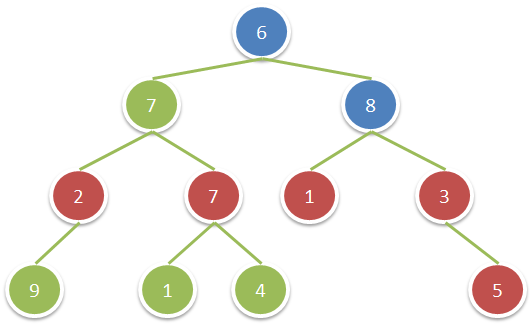# LeetCode 1315. Sum of Nodes with Even-Valued Grandparent

Given a binary tree, return the sum of values of nodes with even-valued grandparent. (A grandparent of a node is the parent of its parent, if it exists.)

If there are no nodes with an even-valued grandparent, return 0.

Example 1:Input: root = [6,7,8,2,7,1,3,9,null,1,4,null,null,null,5]
Output: 18
Explanation: The red nodes are the nodes with even-value grandparent while the blue nodes are the even-value grandparents.

Constraints:

The number of nodes in the tree is between 1 and 10^4.
The value of nodes is between 1 and 100.

 12345678910111213141516171819202122232425262728293031323334 /**  * Definition for a binary tree node.  * struct TreeNode {  *     int val;  *     struct TreeNode *left;  *     struct TreeNode *right;  * };  */ void sumEvenGrandparentCheck(struct TreeNode* root, struct TreeNode* parent, int* sum){     if(root==NULL)return;     if((parent->val&1)==0){         if(root->left){             *sum += root->left->val;         }         if(root->right){             *sum += root->right->val;         }     }     if(root->left){         sumEvenGrandparentCheck(root->left, root, sum);     }     if(root->right){         sumEvenGrandparentCheck(root->right, root, sum);     } } int sumEvenGrandparent(struct TreeNode* root){     if(root==NULL)return 0;     int sum = 0;     sumEvenGrandparentCheck(root->left, root, &sum);     sumEvenGrandparentCheck(root->right, root, &sum);     return sum; }